# Wolstenholme's theorem

## Statement

### Statement in terms of near-central binomial coefficients

Supposeis a prime number greater than 3. Then, we have the following congruence condition on the binomial coefficient: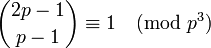### Statement in terms of prime cancellation from binomial coefficient

Supposeis a prime number greater than 3. Then, we have the following congruence condition: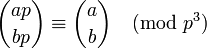This version is due to Glaisher and is similar in structure to Lucas' theorem.

### Statement in terms of generalized harmonic numbers

The following two congruences hold: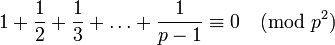and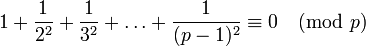What we mean by this is that if we simplify the expressions on the left side and bring them into fractions in reduced form, the numerators are divisible by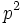andrespectively.